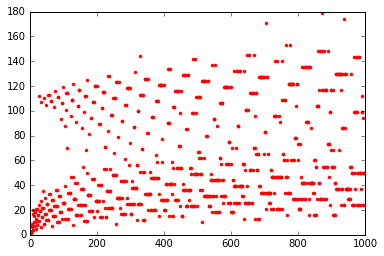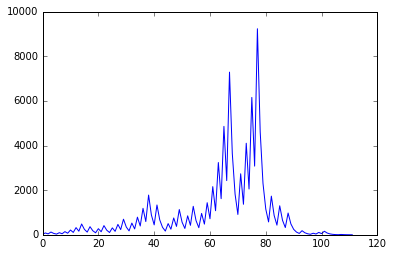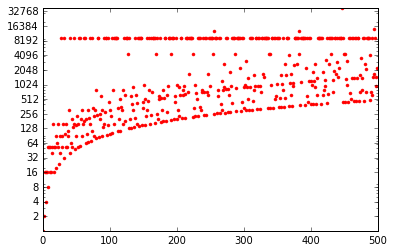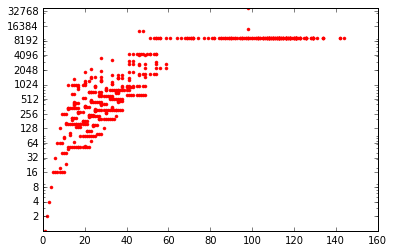# List comprehensions in Python

6 December 2016

Python offers a convenient way to handle iterative structures (such as lists, set or dictionaries).
It is considered good practice to be able to manipulate them fluently. Let’s have a look why!

You can get the original notebook here.

In Python 3, the range keyword does not provide a list but a different structure that can easily be transformed into a list.

range(10)

range(0, 10)

type(range(10)) # this is not a list!

range

list(range(10)) # but you can make a list out of it

[0, 1, 2, 3, 4, 5, 6, 7, 8, 9]


The key point about this notation is performance: when you write range(10000), you do not create a list of size 10000, but a structure that will give you a new integer each time you go through a loop.

In that perspective, you may be comfortable with the following snippet of code:

for i in range(10):
print (i, end=" ")

0 1 2 3 4 5 6 7 8 9


Now, let’s say we want to compute $x \mapsto 2\cdot x$ for each element of a given list (resp. range).
Let us compare the two following ways of writing it.

a = range(1000000)

%%time
new_list = [2*x for x in a]

CPU times: user 92 ms, sys: 16 ms, total: 108 ms
Wall time: 113 ms

%%time
new_list = []
for x in a:
new_list.append(x)

CPU times: user 172 ms, sys: 36 ms, total: 208 ms
Wall time: 207 ms

Definition:
Any syntax going like [f(x) for x in array] is referred to as list comprehension.
Important note: Besides being a more compact way to write things, list comprehension is also more efficient.

The official docs.python.org mentions it quickly here but let’s go through this more into details.

## List comprehensions are not only about lists

List comprehensions are based on a Python specific data structure called a generator.
You can manipulate a generator with brackets (no bracket results in a SyntaxError).

g = (str(i) for i in range(10))
type(g)

generator


You can construct any data structure that accept iterable structures with a generator.

list(g)

['0', '1', '2', '3', '4', '5', '6', '7', '8', '9']


Note that the generator is now empty. You cannot build a second list from the same g:

list(g)

[]


So let’s go through different constructions:

• the regular Python list:
# equivalent notations for lists
list(str(i) for i in range(10))
[str(i) for i in range(10)]

['0', '1', '2', '3', '4', '5', '6', '7', '8', '9']

• the regular Python set:
# equivalent notations for sets
set(str(i) for i in range(10))
{str(i) for i in range(10)}

{'0', '1', '2', '3', '4', '5', '6', '7', '8', '9'}

• even dictionaries (keyword dict) with the column character :
{i: str(i) for i in range(10)}

{0: '0',
1: '1',
2: '2',
3: '3',
4: '4',
5: '5',
6: '6',
7: '7',
8: '8',
9: '9'}


There are actually other useful constructions, e.g. sorted that builds a sorted list from a generator:

[i*(-1)**(i) for i in range(10)]

[0, -1, 2, -3, 4, -5, 6, -7, 8, -9]

sorted(i*(-1)**(i) for i in range(10))

[-9, -7, -5, -3, -1, 0, 2, 4, 6, 8]


Building a set from the generator can also be useful as reflected on the following snippet:

[i**2 for i in range(-5, 5)]

[25, 16, 9, 4, 1, 0, 1, 4, 9, 16]

{i**2 for i in range(-5, 5)}

{0, 1, 4, 9, 16, 25}


You can also build there structures with more complex constructions.
Recall the enumerate and/or zip constructions:

french = ["un", "deux", "trois", "cat", "sank"]
english = ["one", "two", "three", "four", "five"]
words = { i+1: f for i, f in enumerate(zip(french, english)) }
words

{1: ('un', 'one'),
2: ('deux', 'two'),
3: ('trois', 'three'),
4: ('cat', 'four'),
5: ('sank', 'five')}


Looping on a dictionary iterates on the keys:

[str(s) for s in words]

['1', '2', '3', '4', '5']


But you can also use the dict.items() method.
Here is an example to show there is “no” limit in how you can use list comprehensions.

[[key, value, len(value), value.upper(), len(value)]
for key, value in words.items()]

[[1, 'un', 2, 'ONE', 3],
[2, 'deux', 4, 'TWO', 3],
[3, 'trois', 5, 'THREE', 5],
[4, 'cat', 3, 'FOUR', 4],
[5, 'sank', 4, 'FIVE', 4]]


## Common loop patterns using list comprehensions

Check the difference between a zip and a double loop construction:

list(zip(french, english))

[('un', 'one'),
('deux', 'two'),
('trois', 'three'),
('cat', 'four'),
('sank', 'five')]

[(x, y) for x in french for y in english]

[('un', 'one'),
('un', 'two'),
('un', 'three'),
('un', 'four'),
('un', 'five'),
('deux', 'one'),
('deux', 'two'),
('deux', 'three'),
('deux', 'four'),
('deux', 'five'),
('trois', 'one'),
('trois', 'two'),
('trois', 'three'),
('trois', 'four'),
('trois', 'five'),
('cat', 'one'),
('cat', 'two'),
('cat', 'three'),
('cat', 'four'),
('cat', 'five'),
('sank', 'one'),
('sank', 'two'),
('sank', 'three'),
('sank', 'four'),
('sank', 'five')]

Important note: Be aware of which of the two for loops iterate faster

You can also add conditions withing the list comprehension:

[a for a in range(10) if a % 2 == 0]

[0, 2, 4, 6, 8]

Exercice: Use list comprehension to generate a list of (a, b, c) integers such that $$a^2 + b^2 = c^2$$
[ (a, b, c) for a in range(1, 30)
for b in range(a, 30)
for c in range(b, 30) if a**2 + b**2 == c**2 ]

[(3, 4, 5),
(5, 12, 13),
(6, 8, 10),
(7, 24, 25),
(8, 15, 17),
(9, 12, 15),
(10, 24, 26),
(12, 16, 20),
(15, 20, 25),
(20, 21, 29)]


Note that you can also use nested list comprehensions.

[[(x, y) for x in french] for y in english]

[[('un', 'one'),
('deux', 'one'),
('trois', 'one'),
('cat', 'one'),
('sank', 'one')],
[('un', 'two'),
('deux', 'two'),
('trois', 'two'),
('cat', 'two'),
('sank', 'two')],
[('un', 'three'),
('deux', 'three'),
('trois', 'three'),
('cat', 'three'),
('sank', 'three')],
[('un', 'four'),
('deux', 'four'),
('trois', 'four'),
('cat', 'four'),
('sank', 'four')],
[('un', 'five'),
('deux', 'five'),
('trois', 'five'),
('cat', 'five'),
('sank', 'five')]]


## Similar functions in numpy

Python loops are known to be expensive. List comprehensions help, but numpy takes a different approach by unfolding the loops using code written in C.

import numpy as np

%%time
new_list = [2*x for x in range(1000000)]

CPU times: user 84 ms, sys: 32 ms, total: 116 ms
Wall time: 119 ms

a = np.arange(1000000)

%%time
new_list = 2 * a

CPU times: user 20 ms, sys: 0 ns, total: 20 ms
Wall time: 21.9 ms

Important note: Although you cannot write as much in numpy as you can with list comprehension (because of the combination with the if construct), numpy remains the faster option.
• Nested comprehension may remind you the meshgrid function:
z = [[ (x+y) for x in range(10)] for y in range(5)]
z

[[0, 1, 2, 3, 4, 5, 6, 7, 8, 9],
[1, 2, 3, 4, 5, 6, 7, 8, 9, 10],
[2, 3, 4, 5, 6, 7, 8, 9, 10, 11],
[3, 4, 5, 6, 7, 8, 9, 10, 11, 12],
[4, 5, 6, 7, 8, 9, 10, 11, 12, 13]]

a, b = np.meshgrid(np.arange(10), np.arange(5))
c = a + b
c

array([[ 0,  1,  2,  3,  4,  5,  6,  7,  8,  9],
[ 1,  2,  3,  4,  5,  6,  7,  8,  9, 10],
[ 2,  3,  4,  5,  6,  7,  8,  9, 10, 11],
[ 3,  4,  5,  6,  7,  8,  9, 10, 11, 12],
[ 4,  5,  6,  7,  8,  9, 10, 11, 12, 13]])

• You can go through elements of a 2D array with the following constructs:
[col for col in z]

[1, 2, 3, 4, 5]

c[:, 1]

array([1, 2, 3, 4, 5])


Be careful though that numpy does not provide you a fresh copy of the array even if you create intermediate references.

d = c[:, 0]
d *= 0
c

array([[ 0,  1,  2,  3,  4,  5,  6,  7,  8,  9],
[ 0,  2,  3,  4,  5,  6,  7,  8,  9, 10],
[ 0,  3,  4,  5,  6,  7,  8,  9, 10, 11],
[ 0,  4,  5,  6,  7,  8,  9, 10, 11, 12],
[ 0,  5,  6,  7,  8,  9, 10, 11, 12, 13]])


## Yet another implementation for this old academic problem

Say we want to get all the prime numbers lesser than n.
List comprehension can be a comfortable way to compute the sieve of Eratosthenes.

%%timeit
# First compute the non prime numbers
nope = [j for i in range(2, 8) for j in range(i*2, 50, i)]
# Then build a new list not containing prime numbers
[x for x in range(2, 50) if x not in nope]

10000 loops, best of 3: 33.9 µs per loop


Actually the following implementation with sets may be more space and time efficient:

%%timeit
sieve = set(range(2, 50))
for i in range(2, 8):
sieve -= set(range(2*i, 50, i))
sieve

100000 loops, best of 3: 9.82 µs per loop


## Another way to build your own generators

Let’s recall the types of the structures we mentioned:

type(range(10))

range

type(i for i in range(10))

generator


Python provides a yield keyword in order to let you write your own generator objects.
When the program hits a yield keyword:

1. it yields the current value,
2. then waits for the next iteration in a loop (see the __next__ keyword),
3. then re-enters the program where it was last interrupted.

The Syracuse suite is a good study case for this programming style.
See in the doctest how we build the suite using the list constructor on a generator structure.

def syracuse(n):
"""Computes the Syracuse suite.

>>> list(p for p in syracuse(28))
[28, 14, 7, 22, 11, 34, 17, 52, 26, 13, 40, 20, 10, 5, 16, 8, 4, 2, 1]
"""
assert n > 0
yield n
while n != 1:
if n & 1 == 0:
n = n // 2
else:
n = 3 * n + 1
yield n


An interesting plot to draw is the length of the Syracuse list for all integers within a certain range. See how confortable it can be to write it.

%matplotlib inline
import matplotlib.pyplot as plt

def length(iterable):
"""Length of a generator.

The len keyword does not apply on generators.
This makes the trick without expanding the list!
"""
return sum(1 for _ in iterable)

# Note how we can pass a range to plt.plot, not necessarily a list
# Note the multi-level generator in the second part of the array
interval = range(1, 1000)
plt.plot(interval, [length(syracuse(i)) for i in interval], "r.")[i for i in range(1, 50) if length(syracuse(i)) > 100]

[27, 31, 41, 47]


27 is the first integer when the Syracuse suite length exceeds 100.
We can pretty-print the suite with another kind of list comprehension.

print(" ⇢ ".join(str(i) for i in syracuse(27)))

27 ⇢ 82 ⇢ 41 ⇢ 124 ⇢ 62 ⇢ 31 ⇢ 94 ⇢ 47 ⇢ 142 ⇢ 71 ⇢ 214 ⇢ 107 ⇢ 322 ⇢ 161 ⇢
484 ⇢ 242 ⇢ 121 ⇢ 364 ⇢ 182 ⇢ 91 ⇢ 274 ⇢ 137 ⇢ 412 ⇢ 206 ⇢ 103 ⇢ 310 ⇢ 155 ⇢
466 ⇢ 233 ⇢ 700 ⇢ 350 ⇢ 175 ⇢ 526 ⇢ 263 ⇢ 790 ⇢ 395 ⇢ 1186 ⇢ 593 ⇢ 1780 ⇢
890 ⇢ 445 ⇢ 1336 ⇢ 668 ⇢ 334 ⇢ 167 ⇢ 502 ⇢ 251 ⇢ 754 ⇢ 377 ⇢ 1132 ⇢ 566 ⇢
283 ⇢ 850 ⇢ 425 ⇢ 1276 ⇢ 638 ⇢ 319 ⇢ 958 ⇢ 479 ⇢ 1438 ⇢ 719 ⇢ 2158 ⇢ 1079 ⇢
3238 ⇢ 1619 ⇢ 4858 ⇢ 2429 ⇢ 7288 ⇢ 3644 ⇢ 1822 ⇢ 911 ⇢ 2734 ⇢ 1367 ⇢ 4102 ⇢
2051 ⇢ 6154 ⇢ 3077 ⇢ 9232 ⇢ 4616 ⇢ 2308 ⇢ 1154 ⇢ 577 ⇢ 1732 ⇢ 866 ⇢ 433 ⇢
1300 ⇢ 650 ⇢ 325 ⇢ 976 ⇢ 488 ⇢ 244 ⇢ 122 ⇢ 61 ⇢ 184 ⇢ 92 ⇢ 46 ⇢ 23 ⇢ 70 ⇢
35 ⇢ 106 ⇢ 53 ⇢ 160 ⇢ 80 ⇢ 40 ⇢ 20 ⇢ 10 ⇢ 5 ⇢ 16 ⇢ 8 ⇢ 4 ⇢ 2 ⇢ 1

plt.plot(list(syracuse(27)))We can see the suite goes up to 9232 (!) before coming back (quite fast) down to [4, 2, 1].
Another interesting plot shows the height of the suite for each integer.

Important note: max is also a construction that accepts a generator as parameter!
ax = plt.gca()
ax.set_yscale('log')
ax.set_yticks([1 << i for i in range(1, 17)])
ax.set_yticklabels([1 << i for i in range(1, 17)])

interval = range(1, 500)
plt.plot(interval, [max(syracuse(i)) for i in interval], "r.")A last interesting graph plots the height of a suite depending on its length.

ax = plt.gca()
ax.set_yscale('log')
ax.set_yticks([1 << i for i in range(1, 17)])
ax.set_yticklabels([1 << i for i in range(1, 17)])

interval = range(1, 500)
plt.plot([length(syracuse(i)) for i in interval],
[max(syracuse(i)) for i in interval], "r.")